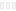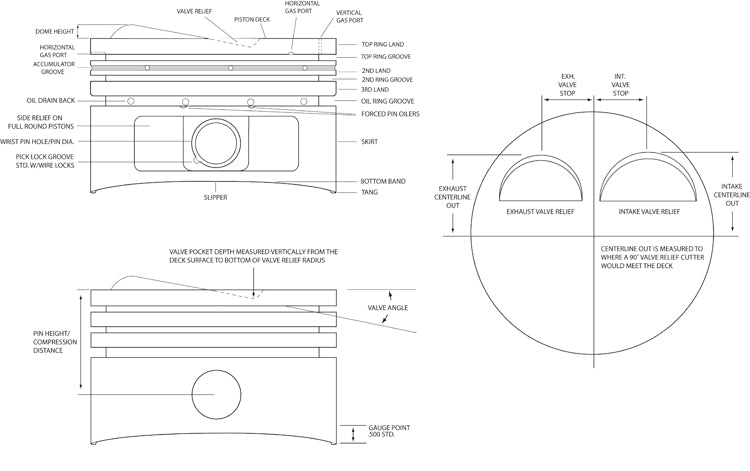CartCall us to order a book!1-800-551-4754

Monday-Friday 8:00am - 4:30pm CST

Calculating Piston Speed

by John Baechtel

Piston speed generally refers to the average or mean speed of the piston as it moves up and down in the cylinder bore during each crankshaft revolution. Since the piston actually comes to a complete stop at the top of the stroke (TDC) and at the bottom of the stroke (BDC), its speed and acceleration at any given point is always changing. The piston is always accelerating from or decelerating to zero speed. The formula for mean piston speed yields an average speed based on two times the stroke (up and down for one revolution), times the engine speed (rpm) divided by 12 to convert to feet per minute (fpm). To simplify the formula, divide the numerator and the denominator by 2.

Piston Speedfpm = stroke x rpm ÷ 6

Let’s work an example for a 302 Ford that has a stroke of 3 inches and a maximum engine speed of 6,000 rpm.

Piston Speedfpm = 3 x 6,000 ÷ 6 = 3,000 fpm

Note that if you did not simplify the formula, the answer still comes out the same. It is the distance the piston travels in one minute. To convert to MPH, multiply the answer by 60 to get feet per hour. Then divide by 5,280 to get miles per hour.

MPH = (mean speed x 60) ÷ feet per mile

MPH = (3,000 x 60) ÷ 5,280 = 34.09 mph

Maximum Piston Speed

You can get a very close approximation of maximum piston speed (ignoring rod center-to-center length and rod angularity) with the following formula. Multiply the stroke by pi and divide by 12 to get feet per revolution. Then multiply by the maximum engine speed to get the maximum feet per minute. This speed occurs about mid stroke, where the connecting rod is ninety degrees to the crankpin. Before that point the piston is accelerating; after it the piston is decelerating. When the piston is exactly at either top or bottom dead center it is stopped and there is no acceleration.

Using the formula for mean piston speed we calculated 3,000 fpm or 34.09 mph for our 302 Ford. Now let’s find the maximum piston speed at 6,000 rpm.

Maximum Piston Speed = MPS

MPSfpm = (stroke x π ÷ 12) x RPM

MPSfpm = (3.00 x 3.14 ÷ 12) x 6,000

MPSfpm = (9.42 ÷ 12) x 6,000 =

.785 x 6,000 = 4,710 fpm

or

MPSfpm x 60 ÷ 5,280 = MPH

4,710 x 60 ÷ 5,280 = 53.52 mph

Mean piston speed has long been used as an indicator of component durability under severe service. It is a good rule for evaluating engine potential and it is even more instructive if you calculate maximum piston speed since one of the axioms of engine performance dictates that power comes from engine speed. The more power strokes per minute, the more power available to do work.

Consider the 2000 Ferrari Formula 1 engine that has a stroke of 1.6-inch. At 18,000 rpm that’s a mean piston speed of 4,800 fpm and a peak speed over 7,536 fpm, or more than 85 mph at mid stroke. Potential disaster for a big-block Chevy, but the Formula 1 engine enjoys the luxury of very exotic materials and very small pistons that have much less mass to accelerate.These piston diagrams illustrate all the important piston features and dimensions. Note in particular the piston pin height, or compression height, which changes according to connecting rod length.